Friday 22nd January 2021

CBSE Guess > Papers > Question Papers > Class XII > 2004 > Maths > Delhi Set-I

MATHS (Set I—Delhi)

SECTION - A

Q. 1. If A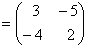,show that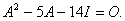Q. 2. Using properties of determinants, solve for x: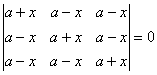Q. 3. An urn contains 7 white, 5 black and 3 red balls. Two balls drawn at random. Find the probability that
(i) Both the bells are red
(ii) One ball is red, the other is black
(iii) One ball is white

Q. 4. A fair die is tossed twice. If the number appearing on the top is less than 3, it is a success.

Q. 5. Evaluate: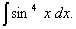Q. 6. Evaluate: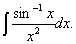Q. 7. Form the differential equation corresponding to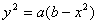where a and b are arbitrary constants.

Q. 8. Solve the differential equation: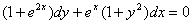Given the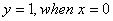Or

Solve the differential equation: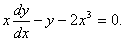Q. 9. Show that in a Boolean algebra, B:
(i)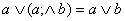(ii)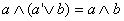Q. 10. Evaluate: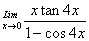Q. 11. Differentiate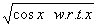from first principles.

Q. 12. Differentiate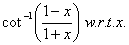Q. 13. Find the equations of the tangent and the normal to the curve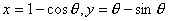at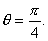Q. 14. Evaluate: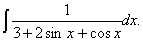Q. 15. Evaluate: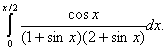Q. 16. Using matrix method solve the following system of linear equations: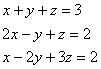Q. 17. Show that a right circular cylinder which is open at the top, and has a given surface area, will have the greatest volume if its height is equal to the radius of its base.

Q. 18. Using integration, find the area of the circle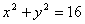which his exterior to the parabola y2 =6x.

Or

Find the area of the smaller region bounded by the cllipse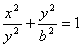and the line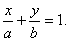SECTION - B

Q. 19. If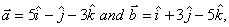then show that the vectors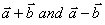are orthogonal.

Or

Find x such that the four points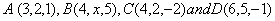are coplanar.

Q. 21. Find the Cartesian and vector equations of a line which passes through the point (1, 2, 3,) and is parallel to the line.

Q. 22. Find the Cartesian equation of the sphere which has the points A (2, -3, 4) and B (-5, 6, -7) as the end points of done of its diameters, also find its centre and radius.

Q. 23. Show that the lines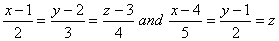intersect. Find the point of intersection also.

Q. 24. Three forces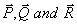acting on a point are in equilibrium. If the angle between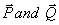and be double the angle between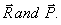prove that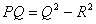Q. 25. Two unlike parallel forces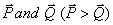act at two points units apart. Show that if the direction of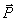be reversed, the resultant will be displaced by a distance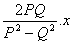units.

Q . 26. A particle starting with some initial velocity and moving with uniform acceleration acquires a velocity of 20 cm/sec after moving through 10 cm from a point P to Q. and a velocity of 30 cm/sec after further moving 20 cm from Q to R in the same direction. Find
(i) its velocity at the point P.
(ii) its acceleration.
(iii) The time it will take and the distance

Or

From a point on the ground at a distance from the foot of a vertical wall, a ball is thrown at an angle of 450 which just clears the top of the wall and afterwards strikes the ground at a distance of y on the other side of the wall. Find the height of the wail.

SECTION - C

Q. 19. Find the banker's discount and true discount on a bill of Rs. 22,800 due 4 months hence at 4% per annum.

Q. 20. A still of exchange drawn on January 4,2003 at 5 months date was discounted on march 26,2003 at 3% per annum. If the banker's discount is Rs.1207.20. find the face value of the bill.

Q. 21. Three urns A, B and C contain 6 red and 4 white: 2 red and 6 white: and 1 red and 5 white balls respectively. An urn is chosen at random and a ball is drawn. If the ball drawn is found to be red. Find the probability that the ball was drawn from urn A.

Q. 22. The mean and variance of a binomial distribution are 4 and 4/3 respectively.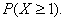Or

If the sum of the mean and variance of a binomial distribution for 5 trials be 1.8, find the distribution.

Q. 23. A, B and C are partners in a business A, being a working partner, receives 10% of the total profit as salary. The remaining profit is distributed among them in the ratio of 2 : 3 : 4. If A gets Rs. 3,00,000 in all, find the shares of B and C.

Q. 24. Find the present value of an annuity of Rs. 1,200 payable at the end of each 6 months for 3 years, when the interest is 8% per annum, compounded semi-annually.Q. 25. The total cost and demand function of an item are given by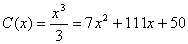and p=100-x respectively.
Write the total revenue function and the profit function. Find the number of items when the profit will be maximum. Find the maximum profit also.

Q. 26. An oil company requires 13,000, 20,000 and 15,000 barrels of high grade, medium grade and low grade oil respectively. Refinery A. produces 100,300 and 200 barrels per day of high, medium and low grade oil respectively whereas the Refinery B produces 200,400 and 100 barrels per day respectively. If A costs Rs. 400 per day and B costs Rs. 300 per day to operate, bow many days should each be run to minimise the cost of requirement?

Or

A firm makes items A and B and the total number of items it can make in a day is 24. It takes one hour to make an item of A and only half an hour to make an item of B. The maximum time available per day is 16 hours. The profit on an item of A is Rs. 300 and on one item of Rs. 160 How many items of each type should be produced to maximise the profit? Solve the problem graphically.

 Maths 2004 Question Papers Class XII Delhi Outside Delhi Compartment Delhi Compartment Outside DelhiSet ISet ISet ISet ISet IISet IISet IISet IISet IIISet III

CBSE 2004 Question Papers Class XII